## Mathematical analysis of Bear Resemblance game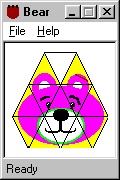• This puzzle can be solved with a set of key maneuvers
• A key maneuver is a sequence of tile movements that result in the exchange of a small number of tiles.
• A maneuver, in the general sense, implies transforming any formation into any other formation.
• A more restrictive maneuver, which we will call an operation transforms a canonical formation into another canonical formation.
• A canonical formation is a formation of the Bear Resemblance game in which the center slot is empty. (see Figure 1)
•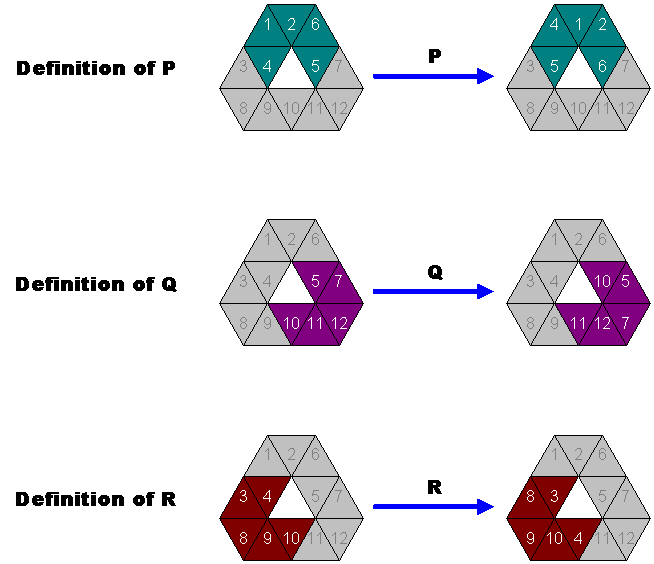Figure 1

• Elementary operations P, Q, and R, are operations consisting of clockwise rotation of 5 tiles (see Figure 1).
• These are short-hand notations for a set of smaller maneuvers. For example, operation P is executed in 6 steps (see Figure 2).
•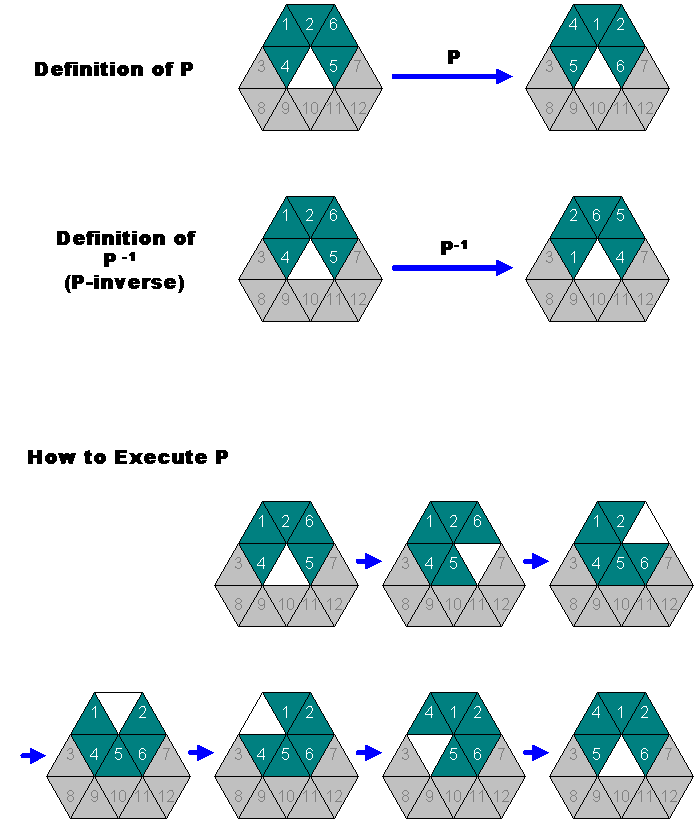Figure 2

• An inverse operation is an operation that cancels out the original operation.
• An inverse operation is denoted by the power of negative 1 (e.g. inverse of P is P^-1). (See Figure 2)
• An inverse operation can be performed by (but not limited to) executing a maneuver backwards.
• A composite operation is two or more operations performed in sequence.
• A composite operation is denoted by juxtaposition of operations, and is evaluated from left to right. For example, AB denotes first performing A, then B.
• Identical operations performed in sequence n times is denoted by the n-th power. For example, A^2 is the same as AA.
• We can call a composite operation an element and give it a name.
• For example P, P2, P3, P4, P5, P6 can be called p1, p2, p3, p4, p5, and p6.
• We can call p5 an identity element and denote it by the symbol e because it brings the tiles back into the same position, resulting in no exchange of tiles.
• We can eliminate the symbol p6 since it is equivalent to p1, i.e., the end result is the same as performing p1.
• The elements {e, p1, p2, p3, p4} form a group of order 5 under the operation of composition.
• This group is isomorphic to Z5, which is the group formed by integer addition under modulo 5 arithmetic.
• P, Q, R, and all other composite operations thereof constitute an exchange group of a larger order.
• The composite operation PQ-1P-1Q results in the rotation of tiles 5, 6, and 7. (see Figure 3). This is the first key operation.
•Figure 3

• Other key operations can be expressed in the same manner.
• For example P-1QP2Q-1R-1P-1R is the second QP-1Q-1R-1P2RP-1 is the third key operation.
• We can combine key operations to form other key operations, which will come in handy when we solve this puzzle.
• We will construct a group using the second and third key operation and study its properties.
• We can think of Bear Resemblance game as a plastic container that has 13 slots containing 12 tiles.
• We name the slots S0, S1, .. S12 from left to right, from top to bottom.
• Let Sm ® Sn denote the act of moving the content of slot m into slot n.
• Let s denote a permutation, i.e., movements of a set of tiles among a closed group of slots.
• For example, s : S0 ® S0, S2 ® S6, S4 ® S2, S6 ® S4 is a permutation of 3 tiles within 4 slots.
• We use the symbols f and g for the second and third key operations.
• We can generate more elements by multiplying f and g arbitrary number of times.
• For example, if we first perform f and then perform g, this is f times g, or fg, and it is a distinct element because looking at the permutation, we can tell that it is not equal to either f or g.
• There are 12 total unique elements that can be generated this way: e, f, g, f2, g2, fg, gf, gf2,f2g, fg2, g2f, and fg2f.

•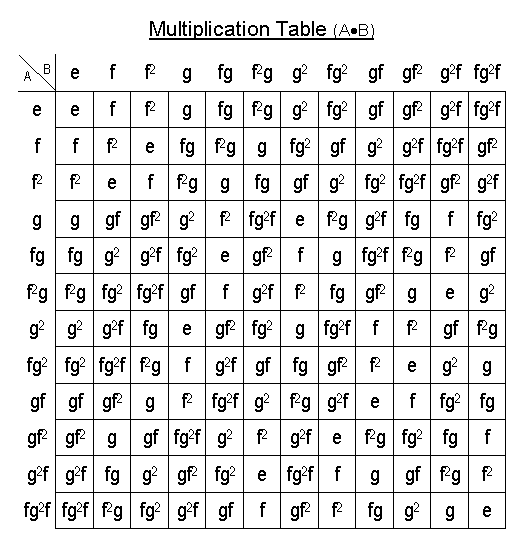Figure 4
• Figure 4 is a multiplication table.
• Figure 5 is a directed graph showing how a new element is generated by multiplication with f or g.
•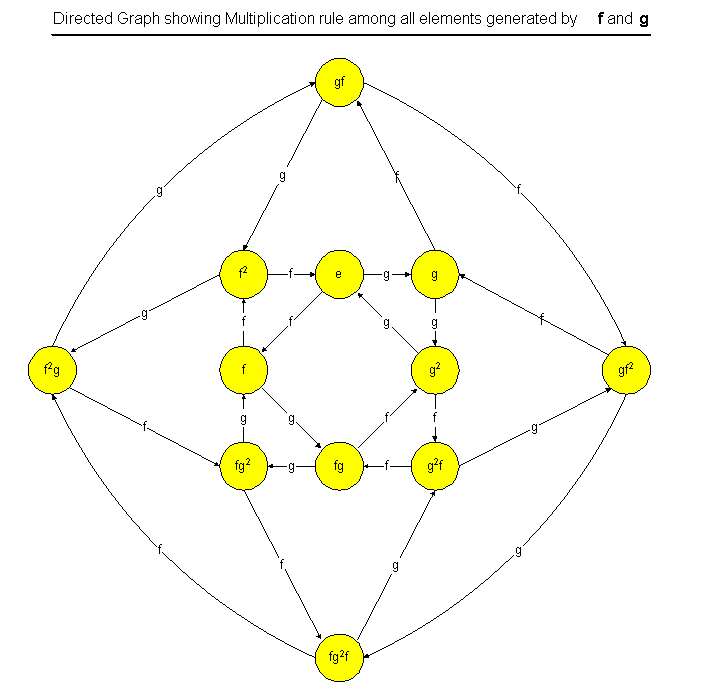Figure 5

• Since the graph is closed, it shows all elements that can be possibly generated.
• Figure 6 is a 3-dimentional representaion, emphasizing the symmetrical nature of this graph.
•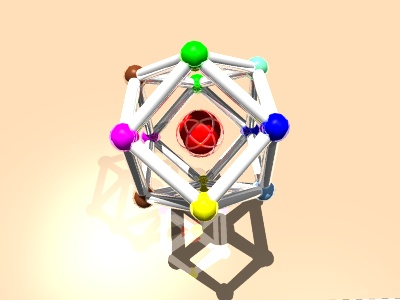Figure 6